Figures index

Structural Performance Assessment of Through-Column-Type Beam-to-Column Joints

American Journal of Civil Engineering and Architecture. 2018, 6(4), 158-167 doi:10.12691/ajcea-6-4-5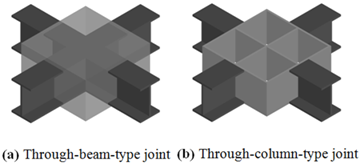• Figure 1. Beam-to-column joints used in RCS frames• Figure 2. Through-column-type joints with one and two transverse stiffeners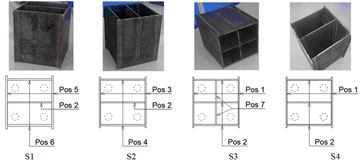• Figure 3. Details of beam-to-column joints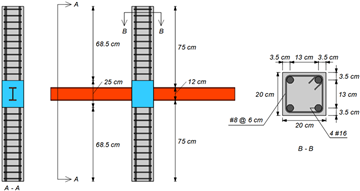• Figure 4. Details of a typical test specimen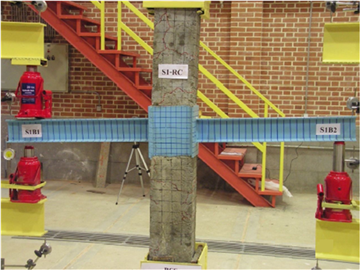• Figure 5. Test setup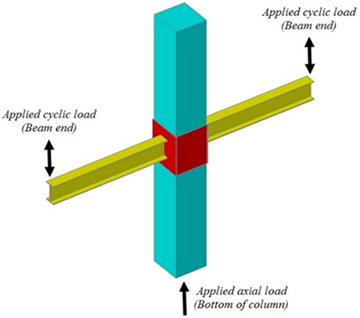• Figure 6. Loading of test specimens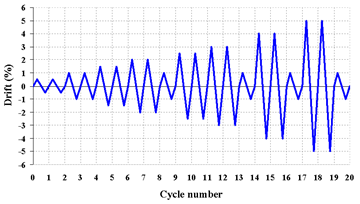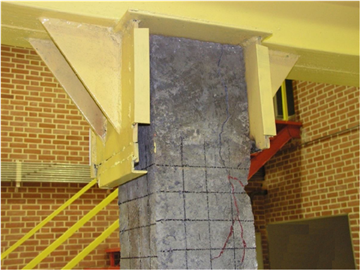• Figure 8. Damage pattern in specimen S1 at 5.0% beam drift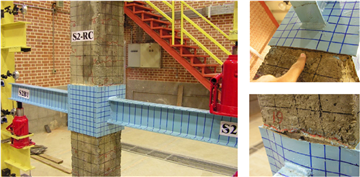• Figure 9. Damage pattern in specimen S2 at 5.0% beam drift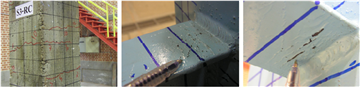• Figure 10. Damage pattern in specimen S3 at 5.0% beam drift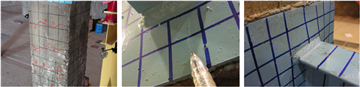• Figure 11. Damage pattern in specimen S4 at 5.0% beam drift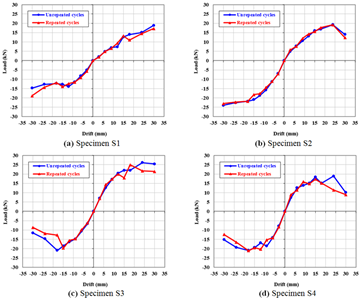• Figure 12. Load-drift curves of the specimens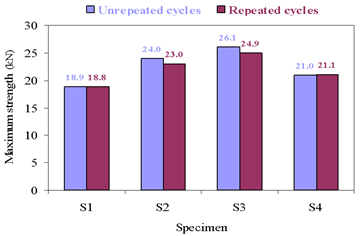• Figure 13. Maximum strengths of the specimens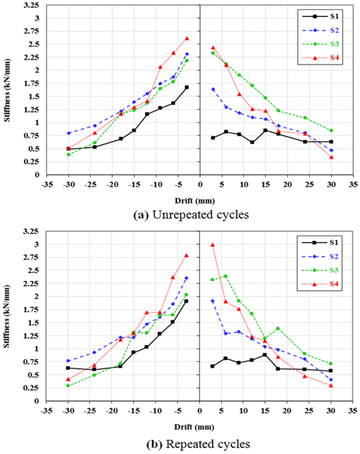• Figure 14. Stiffness performance of the specimens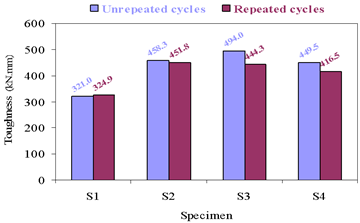• Figure 15. Toughness of the specimens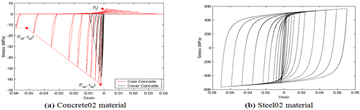• Figure 16. Hysteretic responses of the selected material models in OpenSees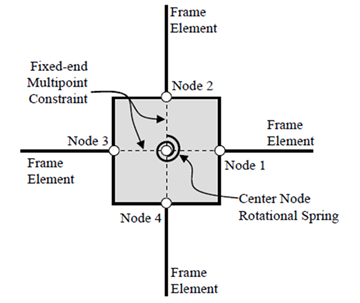• Figure 17. Schematic representation of OpenSees joint element used in this study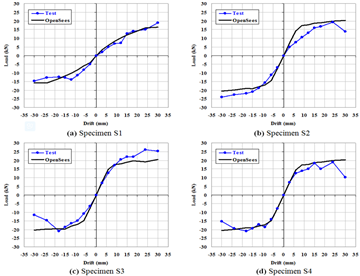• Figure 18. Comparison of test and numerical simulation results (unrepeated cycles)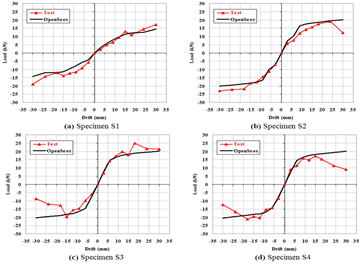• Figure 19. Comparison of test and numerical simulation results (repeated cycles)